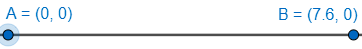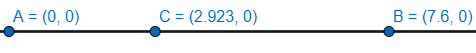Courses
Courses for Kids
Free study material
Free LIVE classes
MoreLIVE
Join Vedantu’s FREE Mastercalss

# To draw a line segment of length 7.6 cm and divide it in ratio 5:8.Verified
360k+ views
Hint: To draw a line segment of length 7.6 cm, we simply have to plot two points (say A and B) which are 7.6 cm apart and then draw a line through them. To divide them in a particular ratio, we make use of properties of ratio and proportions such that if ratio is a:b, one portion of line segment is $\dfrac{b}{a}$times the other segment.

Complete step-by-step solution:
Line segment is a part of a line that is bounded by two distinct end points. Thus, to draw them we are going to take two points A and B. Then we will mark B at a distance of 7.6 cm apart from A and then join them by a line. Thus, to show this,Thus, above shown is a line segment AB which is of 7.6 cm (Since, A and B are 7.6 units (here, units are in cm) apart as shown).
Now to divide the line segment in the ratio of 5:8, we proceed as follows-
Let, there be an internal point C (that is, C is in between A and B), which divides AB in ratio of 5:8. Thus, we would have,
$\dfrac{AC}{CB}=\dfrac{5}{8}$
AC = $\dfrac{5}{8}BC$
Now, AB = AC+CB (apparent from the figure below)
7.6 = $\dfrac{5}{8}BC$+BC
7.6 = $\dfrac{13}{8}BC$
BC = $\dfrac{8\times 7.6}{13}$
BC = $\dfrac{60.8}{13}$
Since, AC = $\dfrac{5}{8}BC$, we have,
AC = $\dfrac{5}{8}\times \dfrac{60.8}{13}$
AC = $\dfrac{304}{104}$
AC = $\dfrac{38}{13}$
Thus, now we can mark point C that divides AB in a ratio of 5:8. We have,Here, AC = $\dfrac{38}{13}$=2.923 cm
Thus, we have point C, which divides AB in the ratio of 5:8.

Note: Another simpler way to proceed with questions involving ratio and proportions (say ratio of a: b) is to assume the proportions to be ak and bk (where k is a constant). So, in this case, the proportions would be 5k and 8k (since, the ratio is 5:8). Now, since, length is 7.6 cm, we have,
5k+8k = 7.6
13k =7.6
k = $\dfrac{7.6}{13}$
Thus, to divide AB by a point C (where C divides AB in ratio 5:8). Thus,
AC = 5k and CB=8k
We have,
AC = $\dfrac{38}{13}$
BC = $\dfrac{60.8}{13}$
Which is the same as the solution above.
Last updated date: 17th Sep 2023
Total views: 360k
Views today: 4.60k